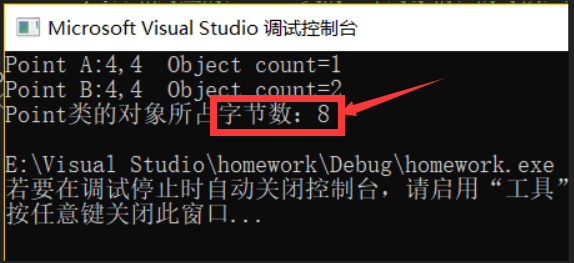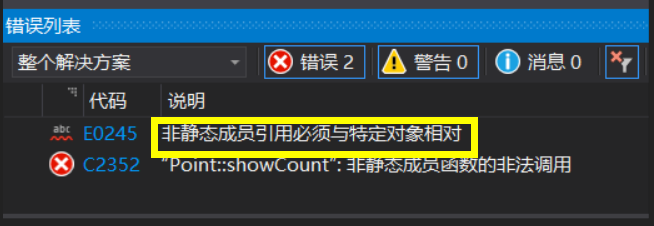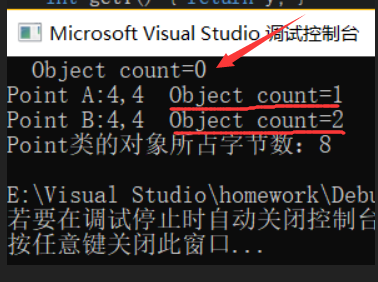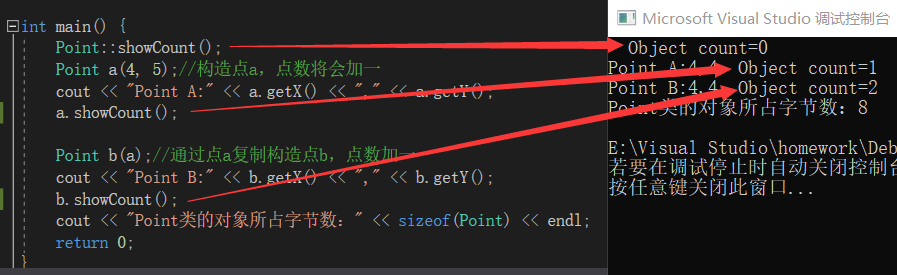# 类的静态成员

## 静态数据成员

#include<iostream>
using namespace std;

class Point{
public:
Point(int x=0,int y=0):x(x),y(x){
//每构造一个点，计数器加一
count++;
}
Point(Point &p){
x=p.x;
y=p.y;
count++;//复制构造函数每被调用一次，计数器加一
}
~Point(){count--;}//相对应的，Point类的一个对象消亡的时候，点数减一
int getX(){return x;}
int getY(){return y;}
void showCount(){
cout<<"  Object count="<<count<<endl;
}
private:
int x,y;
static int count;//静态数据成员，记录点数
};

int Point::count=0;

int main(){
Point a(4,5);//构造点a，点数将会加一
cout<<"Point A:"<<a.getX()<<","<<a.getY();
a.showCount();

Point b(a);//通过点a复制构造点b，点数加一
cout<<"Point B:"<<b.getX()<<","<<b.getY();
b.showCount();
cout<<"Point类的对象所占字节数："<<sizeof(Point)<<endl;//实验观察静态数据成员的属性
return 0;
}• 1、达到了计算点数的功能，创建a点后count的值为1，复制构造点b后count的值为2；
• 2、我通过sizeof运算符得出了Point类的一个对象占有8个字节，但一个对象的数据成员由三个int型的x，y，和count，一个int型的数据所占字节数为4，这三个int型变量之间的不同点在于count是用static定义的静态数据，这就说明了所得的8个字节是x和y的，静态数据count就是所有对象共同维护和使用的数据，这就是计算得出的字节数为8不为12原因。

## 静态函数成员

### 方案一

int main(){
Point::showCount();//希望能够输出点数的初始值
Point a(4,5);//构造点a，点数将会加一
cout<<"Point A:"<<a.getX()<<","<<a.getY();
a.showCount();

Point b(a);//通过点a复制构造点b，点数加一
cout<<"Point B:"<<b.getX()<<","<<b.getY();
b.showCount();
cout<<"Point类的对象所占字节数："<<sizeof(Point)<<endl;//实验观察静态数据成员的属性
return 0;
}### 方案二

stactic void showCount(){
cout<<"  Object count="<<count<<endl;
}


int main(){
Point::showCount();//看是否能输出点数的初始值
Point a(4,5);//构造点a，点数将会加一
cout<<"Point A:"<<a.getX()<<","<<a.getY();
Point::showCount();

Point b(a);//通过点a复制构造点b，点数加一
cout<<"Point B:"<<b.getX()<<","<<b.getY();
Point::showCount();
cout<<"Point类的对象所占字节数："<<sizeof(Point)<<endl;//实验观察静态数据成员的属性
return 0;
}int main(){
Point::showCount();//输出点数的初始值
Point a(4,5);//构造点a，点数将会加一
cout<<"Point A:"<<a.getX()<<","<<a.getY();
a.showCount();//通过对象调用静态函数

Point b(a);//通过点a复制构造点b，点数加一
cout<<"Point B:"<<b.getX()<<","<<b.getY();
b.showCount();//通过对象调用静态函数
cout<<"Point类的对象所占字节数："<<sizeof(Point)<<endl;//实验观察静态数据成员的属性
return 0;posted @ 2019-09-22 15:53  Wybie  阅读(432)  评论(0编辑  收藏  举报